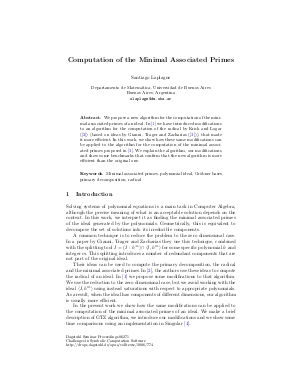Document# Computation of the Minimal Associated Primes

### Author Santiago Laplagne## File

DagSemProc.06271.6.pdf
• Filesize: 170 kB
• 6 pages

## Cite As

Santiago Laplagne. Computation of the Minimal Associated Primes. In Challenges in Symbolic Computation Software. Dagstuhl Seminar Proceedings, Volume 6271, pp. 1-6, Schloss Dagstuhl - Leibniz-Zentrum für Informatik (2006)
https://doi.org/10.4230/DagSemProc.06271.6

## Abstract

Solving systems of polynomial equations is a main task in Computer Algebra, although the precise meaning of what is an acceptable solution depends on the context. In this talk, we interpret it as finding the minimal associated primes of the ideal generated by the polynomials. Geometrically, this is equivalent to decompose the set of solutions into its irreducible components. We study the existing algorithms, and propose some modifications. A common technique used is to reduce the problem to the zero dimensional case. In a paper by Gianni, Trager and Zacharias they use this technique, combined with the splitting tool \$I = (I : h^infty) cap langle I, h^m angle\$ for some specific polynomial \$h\$ and integer \$m\$. This splitting introduces a number of redundant components that are not part of the original ideal. In the algorithm we present here, we use the reduction to the zero dimensional case, but we avoid working with the ideal \$langle I, h^m angle\$. As a result, when the ideal has components of different dimensions, our algorithm is usually more efficient.
##### Keywords
• Minimal associated primes
• groebner basis
• polynomail equations

## Metrics

• Access Statistics
• Total Accesses (updated on a weekly basis)
0